# Fractions - 6th grade (11y) - math problems

#### Number of problems found: 267

• Tennis balls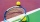Can of tennis balls contains 3 balls per can and cost \$7 how much will it cost for 36 tennis balls?
• From aFrom a 1 meter ribbon, Ericka cut 2/4 meter for her hat and another 1/4 meter for her bag. How long was the remaining piece?2 and 1 8th plus 1 and 1 3rd =7 is added to the sum of 4/5 and 6/7
• One third 2One third of all students in class live in a house. If here are 42 students in a class, how many of them live in house?
• ChestnutsThree divisions of nature protectors participated in the collection of chestnut trees.1. the division harvested 1250 kg, the 2nd division by a fifth more than the 1st division and the 3rd division by a sixth more than the second division. How many tons of
• Candies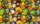There are 150 candies in the bowl. Ivan took 7 thirtieths, Charles 6 fifteenths, and Thomas 3 tenths. How many candies are left in the bowl?
• Empty and fullAn empty can has a mass of 1/6 lb. When it is filled with sand, it has a mass of 7/12 lb. Find the mass of the sand in the can?
• A library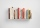A library has 12,500 fiction books and 19,000 non fiction books. Currently 2/5 of the fiction books are checked out. Currently 2/5 of the non fiction books are checked out. Of the books checked out, only 1/10 are due back this week. How many books are due
• Milk cratesA farmer sells milk in crates that hold 15 bottles. She has 34,125 bottles of milk. How many crates can the farmer fill?
• Numbers divisionWith what number should be divided mixed number 2 3/4 to get 11/12?
• Milk2cheese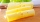From 40 liters of milk is produced 8 kg of cheese how many liters of milk are needed to produce 2 kg of cheese?
• A quotientWhat is the quotient of 3/10 divided by 2/4 as a fraction
• The plan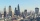The plan of the housing estate is in three scales 1: 5000,1: 10000,1: 15000. The distance between two points on a plan with a scale of 1: 10000 is 12 cm. What is this distance on the other two plans? What is this distance?
• Equation 20In given equation: 8/9-4/5=2/9+x, find x
• Akpan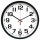Akpan spent 3/8 of his time in school during the week. What fraction of his time does he spend at home during the week?
• Fractions 3Calculate 1/9 of 27:
• Fractions 4How many 2/3s are in 6?
• Cakes1/3 poppy cake, 1/3 apple, 15 pieces of cheese. How many are totally cakes?
• Missing numberBlank +1/6 =3/2 find the missing number

Do you have an interesting mathematical word problem that you can't solve it? Submit a math problem, and we can try to solve it.

We will send a solution to your e-mail address. Solved examples are also published here. Please enter the e-mail correctly and check whether you don't have a full mailbox.

Please do not submit problems from current active competitions such as Mathematical Olympiad, correspondence seminars etc...

Need help calculate sum, simplify or multiply fractions? Try our fraction calculator.